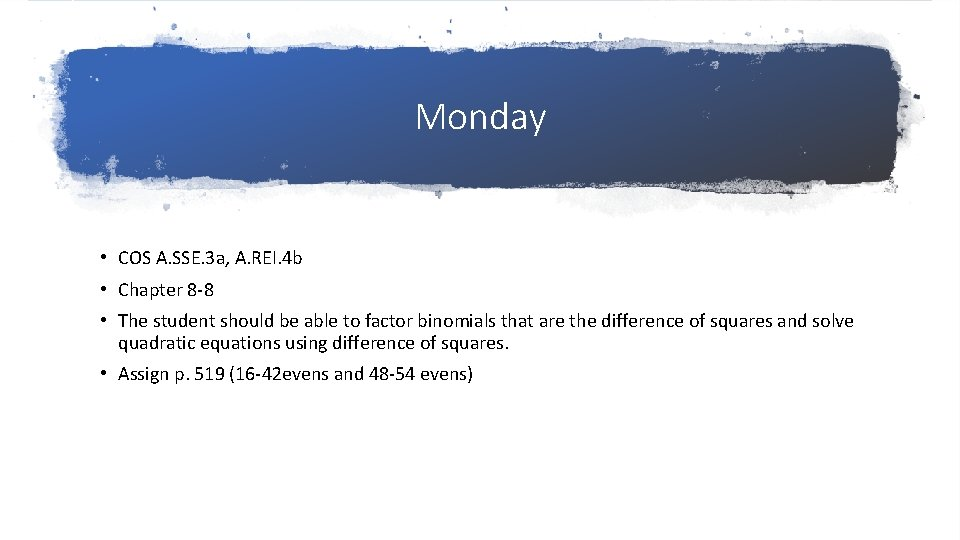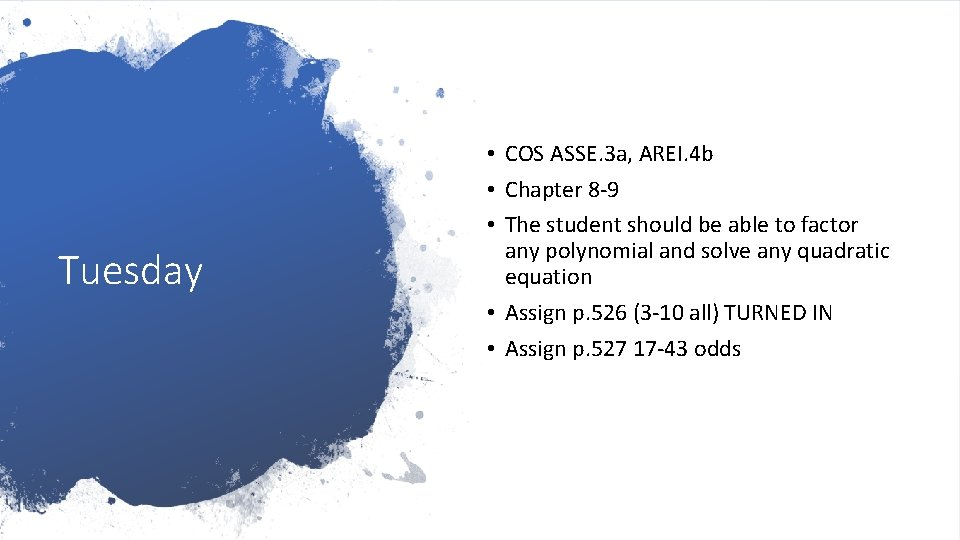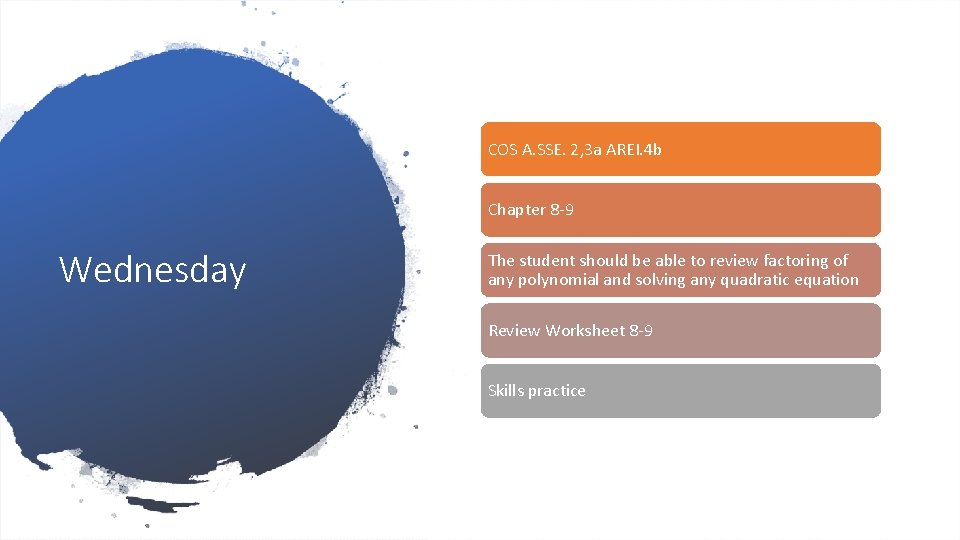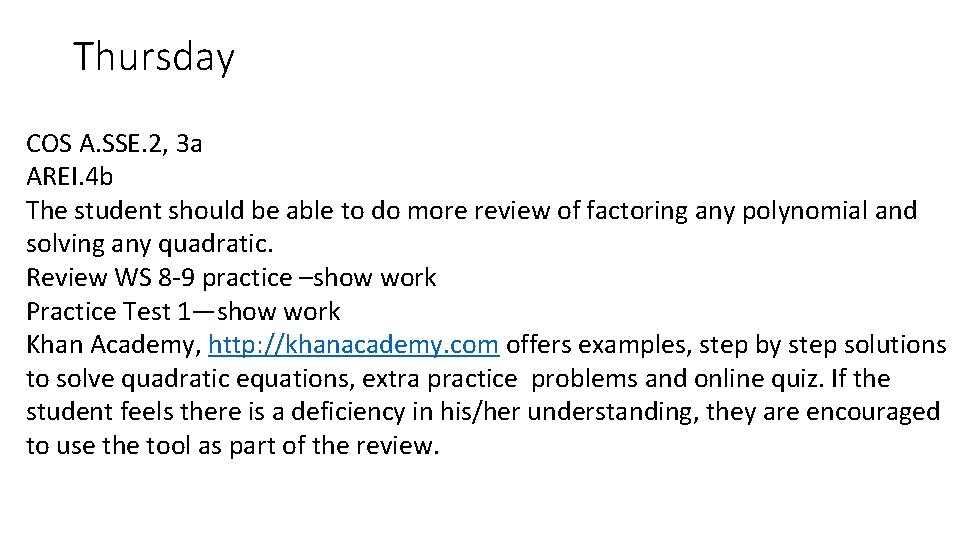# Algebra 1 B Lesson plans 2 nd block

• Slides: 6
Download presentationAlgebra 1 B Lesson plans 2 nd block 4/29 -5/3/2019Monday • COS A. SSE. 3 a, A. REI. 4 b • Chapter 8 -8 • The student should be able to factor binomials that are the difference of squares and solve quadratic equations using difference of squares. • Assign p. 519 (16 -42 evens and 48 -54 evens)Tuesday • COS ASSE. 3 a, AREI. 4 b • Chapter 8 -9 • The student should be able to factor any polynomial and solve any quadratic equation • Assign p. 526 (3 -10 all) TURNED IN • Assign p. 527 17 -43 oddsCOS A. SSE. 2, 3 a AREI. 4 b Chapter 8 -9 Wednesday The student should be able to review factoring of any polynomial and solving any quadratic equation Review Worksheet 8 -9 Skills practiceThursday COS A. SSE. 2, 3 a AREI. 4 b The student should be able to do more review of factoring any polynomial and solving any quadratic. Review WS 8 -9 practice –show work Practice Test 1—show work Khan Academy, http: //khanacademy. com offers examples, step by step solutions to solve quadratic equations, extra practice problems and online quiz. If the student feels there is a deficiency in his/her understanding, they are encouraged to use the tool as part of the review.Friday COS ASSE. 2, 3 a AREI. 4 b Assign practice test 2 SHOW WORK Continue review on http: //Khan. Academy. com The student should be able to do more review of factoring polynomials solving quadratic equations